### Home > PC > Chapter 2 > Lesson 2.3.4 > Problem2-114

2-114.
1. Simplify the following rational expressions. Homework Help ✎

1.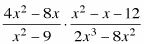2.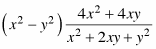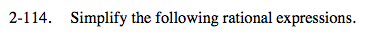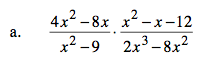Factor all of the expressions. Then simplify.

$\frac{4x(x-2)}{(x-3)(x+3)}\cdot \frac{(x-4)(x+3)}{2x^2(x-4)}$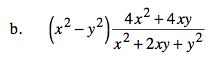Write (x2y2) over 1. Then follow the same process as shown in part (a).